# 16_05.ppt

Nov. 11, 2022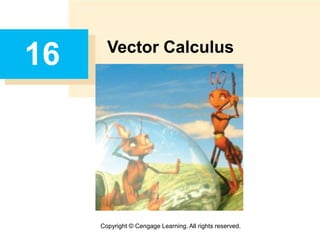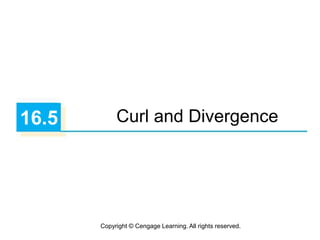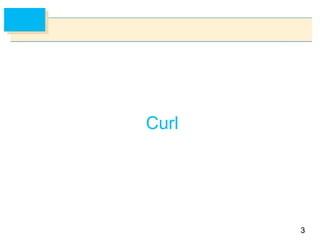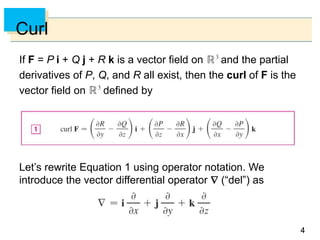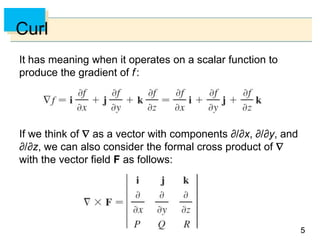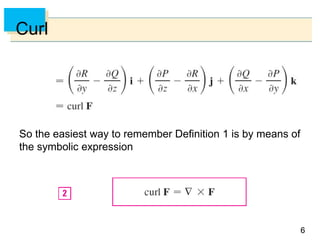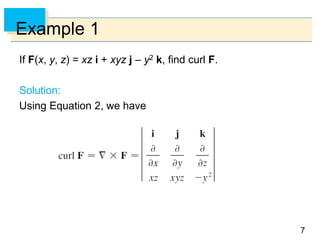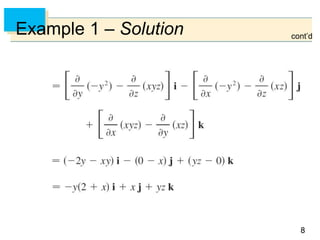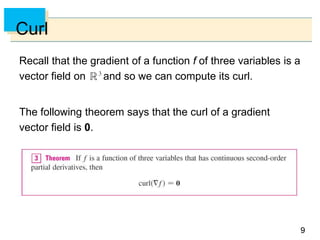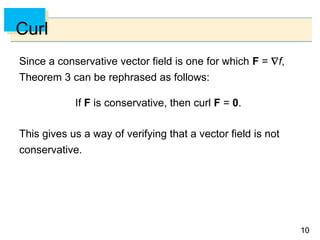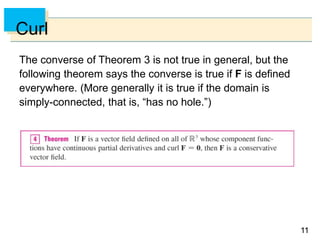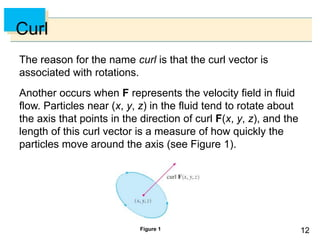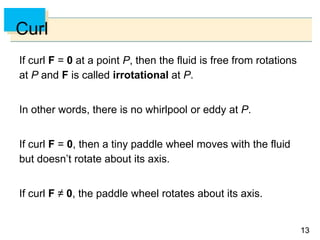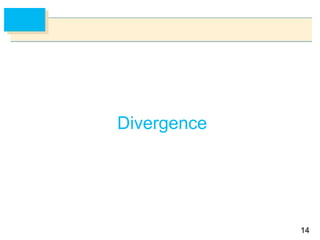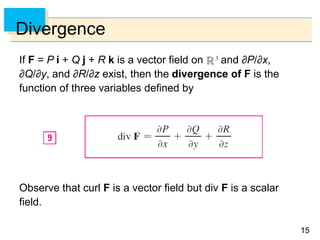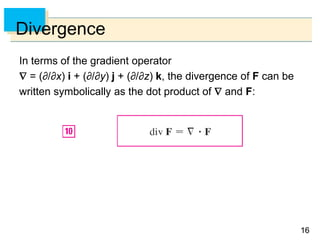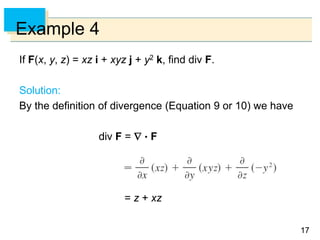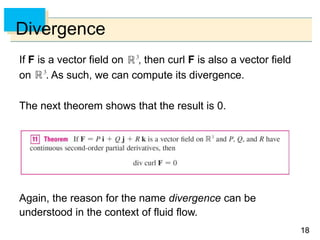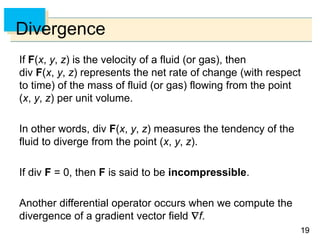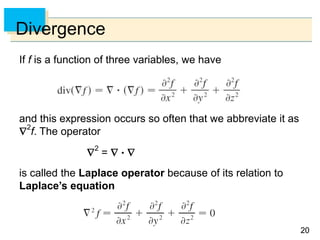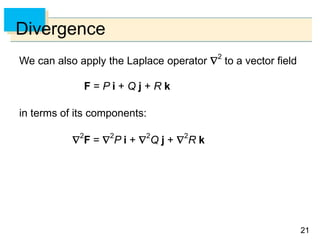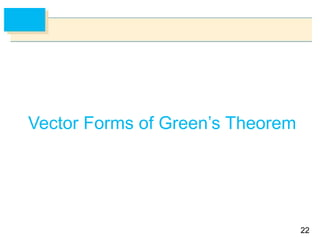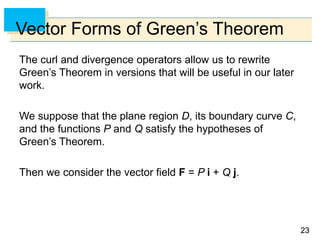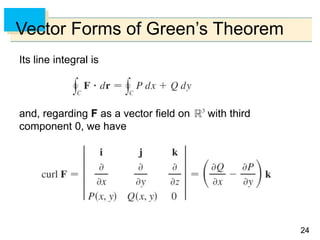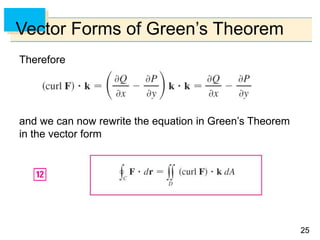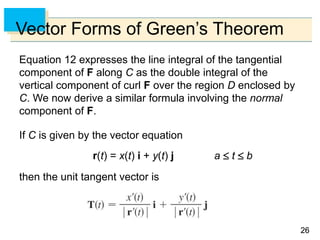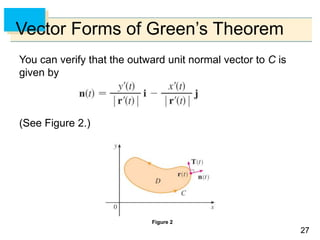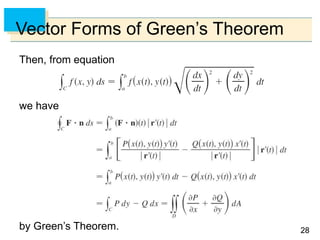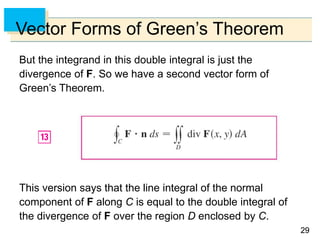1 of 29

### 16_05.ppt

• 4. 4 4 Curl If F = P i + Q j + R k is a vector field on and the partial derivatives of P, Q, and R all exist, then the curl of F is the vector field on defined by Let’s rewrite Equation 1 using operator notation. We introduce the vector differential operator  (“del”) as
• 5. 5 5 Curl It has meaning when it operates on a scalar function to produce the gradient of f: If we think of  as a vector with components ∂/∂x, ∂/∂y, and ∂/∂z, we can also consider the formal cross product of  with the vector field F as follows:
• 6. 6 6 Curl So the easiest way to remember Definition 1 is by means of the symbolic expression
• 7. 7 7 Example 1 If F(x, y, z) = xz i + xyz j – y2 k, find curl F. Solution: Using Equation 2, we have
• 8. 8 8 Example 1 – Solution cont’d
• 9. 9 9 Curl Recall that the gradient of a function f of three variables is a vector field on and so we can compute its curl. The following theorem says that the curl of a gradient vector field is 0.
• 10. 10 10 Curl Since a conservative vector field is one for which F = f, Theorem 3 can be rephrased as follows: If F is conservative, then curl F = 0. This gives us a way of verifying that a vector field is not conservative.
• 11. 11 11 Curl The converse of Theorem 3 is not true in general, but the following theorem says the converse is true if F is defined everywhere. (More generally it is true if the domain is simply-connected, that is, “has no hole.”)
• 12. 12 12 Curl The reason for the name curl is that the curl vector is associated with rotations. Another occurs when F represents the velocity field in fluid flow. Particles near (x, y, z) in the fluid tend to rotate about the axis that points in the direction of curl F(x, y, z), and the length of this curl vector is a measure of how quickly the particles move around the axis (see Figure 1). Figure 1
• 13. 13 13 Curl If curl F = 0 at a point P, then the fluid is free from rotations at P and F is called irrotational at P. In other words, there is no whirlpool or eddy at P. If curl F = 0, then a tiny paddle wheel moves with the fluid but doesn’t rotate about its axis. If curl F ≠ 0, the paddle wheel rotates about its axis.
• 15. 15 15 Divergence If F = P i + Q j + R k is a vector field on and ∂P/∂x, ∂Q/∂y, and ∂R/∂z exist, then the divergence of F is the function of three variables defined by Observe that curl F is a vector field but div F is a scalar field.
• 16. 16 16 Divergence In terms of the gradient operator  = (∂/∂x) i + (∂/∂y) j + (∂/∂z) k, the divergence of F can be written symbolically as the dot product of  and F:
• 17. 17 17 Example 4 If F(x, y, z) = xz i + xyz j + y2 k, find div F. Solution: By the definition of divergence (Equation 9 or 10) we have div F =   F = z + xz
• 18. 18 18 Divergence If F is a vector field on , then curl F is also a vector field on . As such, we can compute its divergence. The next theorem shows that the result is 0. Again, the reason for the name divergence can be understood in the context of fluid flow.
• 19. 19 19 Divergence If F(x, y, z) is the velocity of a fluid (or gas), then div F(x, y, z) represents the net rate of change (with respect to time) of the mass of fluid (or gas) flowing from the point (x, y, z) per unit volume. In other words, div F(x, y, z) measures the tendency of the fluid to diverge from the point (x, y, z). If div F = 0, then F is said to be incompressible. Another differential operator occurs when we compute the divergence of a gradient vector field f.
• 20. 20 20 Divergence If f is a function of three variables, we have and this expression occurs so often that we abbreviate it as 2 f. The operator  2 =    is called the Laplace operator because of its relation to Laplace’s equation
• 21. 21 21 Divergence We can also apply the Laplace operator  2 to a vector field F = P i + Q j + R k in terms of its components:  2 F =  2 P i +  2 Q j +  2 R k
• 22. 22 22 Vector Forms of Green’s Theorem
• 23. 23 23 Vector Forms of Green’s Theorem The curl and divergence operators allow us to rewrite Green’s Theorem in versions that will be useful in our later work. We suppose that the plane region D, its boundary curve C, and the functions P and Q satisfy the hypotheses of Green’s Theorem. Then we consider the vector field F = P i + Q j.
• 24. 24 24 Vector Forms of Green’s Theorem Its line integral is and, regarding F as a vector field on with third component 0, we have
• 25. 25 25 Vector Forms of Green’s Theorem Therefore and we can now rewrite the equation in Green’s Theorem in the vector form
• 26. 26 26 Vector Forms of Green’s Theorem Equation 12 expresses the line integral of the tangential component of F along C as the double integral of the vertical component of curl F over the region D enclosed by C. We now derive a similar formula involving the normal component of F. If C is given by the vector equation r(t) = x(t) i + y(t) j a  t  b then the unit tangent vector is
• 27. 27 27 Vector Forms of Green’s Theorem You can verify that the outward unit normal vector to C is given by (See Figure 2.) Figure 2
• 28. 28 28 Vector Forms of Green’s Theorem Then, from equation we have by Green’s Theorem.
• 29. 29 29 Vector Forms of Green’s Theorem But the integrand in this double integral is just the divergence of F. So we have a second vector form of Green’s Theorem. This version says that the line integral of the normal component of F along C is equal to the double integral of the divergence of F over the region D enclosed by C.